# 如何在Excel中计算年龄

MS Excel，或Excel，是广泛使用的电子表格软件，具有各种内置工具和功能。它帮助我们记录各种数据集并在多个单元格上执行计算。一个经典的计算示例是计算两个日期之间的差值。然而，这听起来不像是一个有用的计算。但是，相同的技术对于在Microsoft Excel中找到或计算某人或某物的年龄有些关键。

## 我们如何在Excel中计算年龄

### 计算年龄（以年为单位）

Excel中的DATEDIF函数是计算一个人年龄的最常用函数。它是一个易于使用、内置的、最合适的函数，将出生日期作为输入日期，返回人的年龄作为输出数据。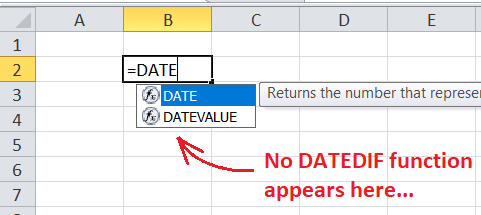DATEDIF函数的通用语法定义如下：

=DATEDIF(start_date, end_date, unit)


• start_date： 此参数用于指定要计算差值结果的起始日期/初始日期的区间。可以以日期、双引号内的文本字符串、序列号或其他函数（如DATE()）的结果形式给出。
• end_date： 此参数用于指定要计算差值结果的结束日期的区间。接受类似于start_date的数据。
• unit： 此参数帮助我们指定DATEDIF返回的结果类型。根据提供的单位类型，我们可以获得六种不同的输出格式。可以输入单位为Y、M、D、MD、YM和YD。其中，Y表示年，M表示月，D表示日。

=DATEDIF(出生日期,特定日期,”Y”)

=DATEDIF(出生日期,TODAY(),”Y”)

=DATEDIF(B2,TODAY(),”Y”)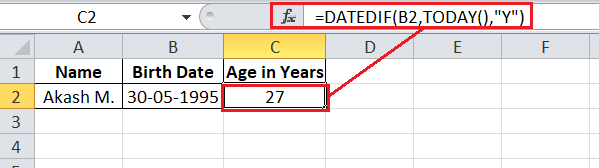=YEARFRAC(Birth_Date,Specific_Date)


=YEARFRAC(Birth_Date,TODAY())

=INT(YEARFRAC(Birth_Date,Specific_Date))

=INT(YEARFRAC(B2,A2))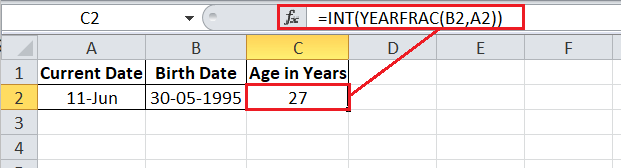=INT(YEARFRAC(B2, TODAY()))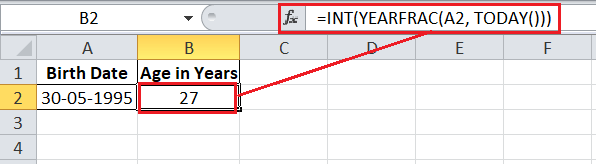=ROUNDDOWN((Specific_Date - Birth_Date)/365.25,0)


ROUNDDOWN公式是计算年龄的一种好方法，但并不完美。假设一个孩子尚未经历过任何闰年，我们使用这个公式通过365.25来计算年龄，那么公式会返回错误的年龄。

=ROUNDDOWN((A2-B2)/365.25,0)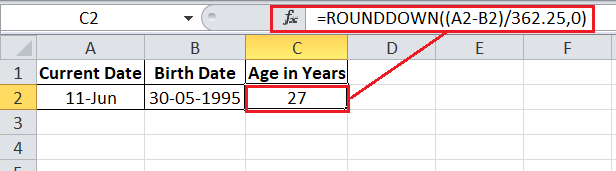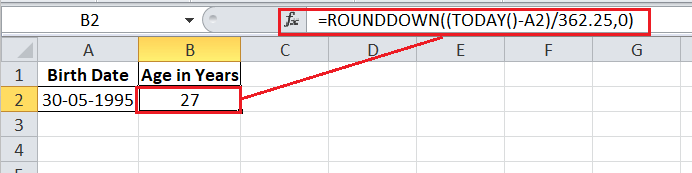=(TODAY()-B2)/365

=INT((TODAY()-B2)/365)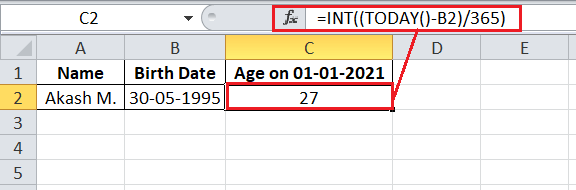### 计算月龄

=DATEDIF(B2,TODAY(),”M”)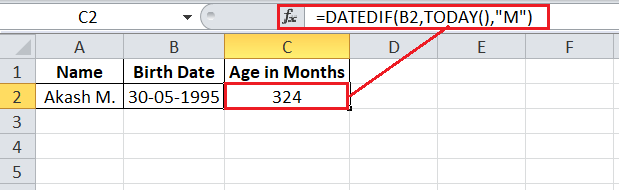### 计算年龄天数

=DATEDIF(B2,TODAY(),”D”)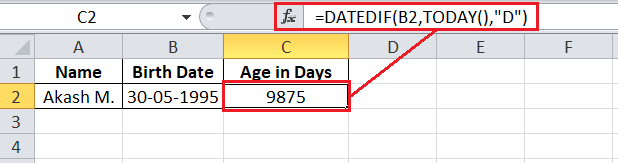### 计算年龄的年，月和日的总和

• 计算完整年数： =DATEDIF(B2,TODAY(),”Y”)
• 计算剩余月数： =DATEDIF(B2,TODAY(),”YM”)
• 计算剩余天数： =DATEDIF(B2,TODAY(),”MD”)

**=DATEDIF(B2,TODAY(),”Y”) &DATEDIF(B2,TODAY(),”YM”)&DATEDIF(B2,TODAY(),”MD”) **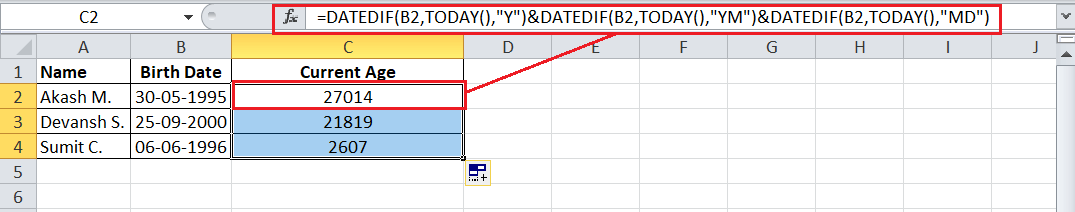=DATEDIF(B2,TODAY(),"Y") & " 年, " & DATEDIF(B2,TODAY(),"YM") & " 月, " & DATEDIF(B2,TODAY(),"MD") & " 天"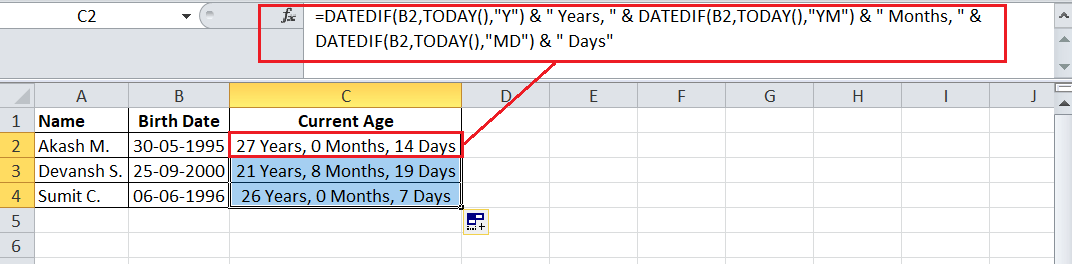=IF(DATEDIF(B2, TODAY(),"Y")=0,"",DATEDIF(B2, TODAY(),"Y") &" Years, ")& IF(DATEDIF(B2, TODAY(),"YM")=0,"",DATEDIF(B2, TODAY(),"YM")&" Months, ")& IF(DATEDIF(B2, TODAY(),"MD")=0,"",DATEDIF(B2, TODAY(),"MD")&" Days")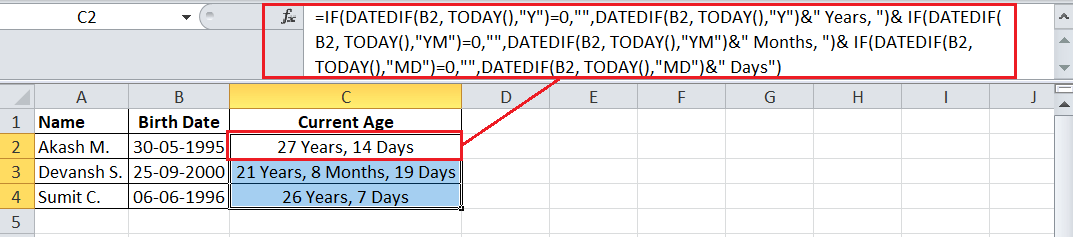### 计算特定日期的年龄

=DATEDIF(出生日期,特定日期,"Y")

=DATEDIF(B2,DATE(2021,1,1),"Y")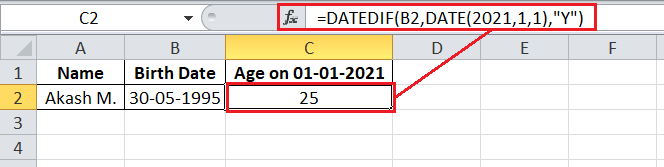=IF(DATEDIF(B2, "1/1/2021","Y")=0,"",DATEDIF(B2, "1/1/2021","Y") &"年, ")& IF(DATEDIF(B2, "1/1/2021","YM")=0,"",DATEDIF(B2, "1/1/2021","YM")&"月, ")& IF(DATEDIF(B2, "1/1/2021","MD")=0,"",DATEDIF(B2, "1/1/2021","MD")&"天")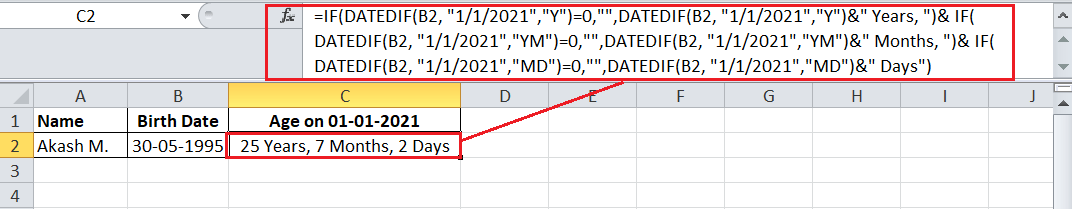=IF(DATEDIF(B2, C2,"Y")=0,"",DATEDIF(B2, C2,"Y") &" Years, ")& IF(DATEDIF(B2, C2,"YM")=0,"",DATEDIF(B2, C2,"YM")&" Months, ")& IF(DATEDIF(B2, C2,"MD")=0,"",DATEDIF(B2, C2,"MD")&" Days")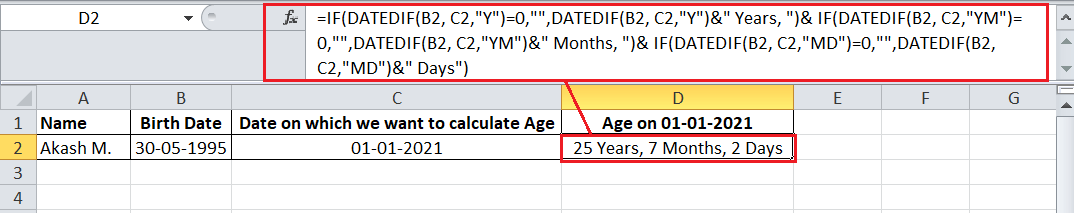• 微信订阅
• 回顶